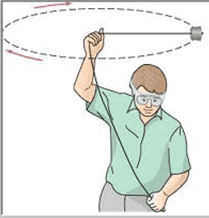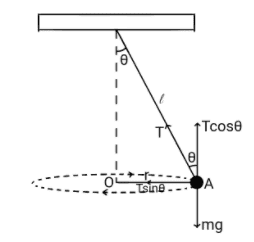# Tension of string acting on stone moving in horizontal circular motion

• songoku

#### songoku

Homework Statement
This is not homework. I just want to know about horizontal circular motion. Please see below
Relevant Equations
Centripetal forceIs it possible for the stone to move in horizontal circular motion just like in the picture? I try to draw the free body diagram of the stone and there are two forces acting on the stone, its weight (directed downwards) and the tension of the string (directed to the left).

The tension will provide the centripetal force needed to move in circle, but there is no force to balance the weight so I think it is not possible for the stone to move in horizontal circular motion like in the picture. Am I correct?

Thanks

You are right. Under influence of gravity conical pendulum motion is the right one.

•songoku
You are right. Under influence of gravity conical pendulum motion is the right one.
Now I want to consider conical pendulumThe equation would be:
$$T \cos \theta=mg$$
$$T \sin \theta=m \frac{v^2}{r}$$

I want to ask how the radius and tension will change if I reduce the speed at which I swing the stone.

If I imagine the motion at lower speed, to maintain horizontal circular motion, the radius will decrease.
Since the radius decreases, ##\theta## will also decrease so tension must decrease to balance the weight.

I get ##\theta## will decrease from the diagram and I get tension has to decrease from equation in vertical direction, but I get radius will decrease only by using imagination. If I want to show the radius needs to decrease because of lower speed through equation, what should I do?

Thanks

Hello, you are right in everything you have deduced.
If you divide member by member or both equations you get a direct relationship between the tangent of the angle, the weight, the velocity and the radius.
and if you use the relation ##\sin ^ 2 \theta + \cos ^ 2 \theta = 1## to eliminate both the sine and the cosine of this system of equations, you will have a quadratic equation to evaluate how the tension changes as a function of weight, speed and radius.

•songoku
Is it possible for the stone to move in horizontal circular motion just like in the picture?
It is moving in a horizontal circle. It is just the string that cannot be horizontal.
If I want to show the radius needs to decrease because of lower speed through equation, what should I do?
You need to use the fact that the string length is constant.

•songoku
If you divide member by member or both equations you get a direct relationship between the tangent of the angle, the weight, the velocity and the radius.
I get
$$v^2=gr \tan \theta$$

and if you use the relation ##\sin ^ 2 \theta + \cos ^ 2 \theta = 1## to eliminate both the sine and the cosine of this system of equations, you will have a quadratic equation to evaluate how the tension changes as a function of weight, speed and radius.
I get:
$$T^2=\frac{m^{2} v^4}{r^2}+m^2 g^2$$

But how to analyze the change in radius if the speed changes from these two equations? Because there are more than 2 variables change in both equations

You need to use the fact that the string length is constant.
I am sorry I don't know how to use your hint. I use string length to analyze the angle (string length is constant means that the angle should decreases when r decreases) but how to relate that to speed?

Thanks

•Delta2
I am sorry I don't know how to use your hint. I use string length to analyze the angle (string length is constant means that the angle should decreases when r decreases) but how to relate that to speed?
In post #3 you have two equations. The relationship between angle, string length and radius is a third. Eliminate T and theta to get an equation relating v to r.

•songoku
But how to analyze the change in radius if the speed changes from these two equations?
The first of the equations ##u^2=gr\tan\theta## tells you that when you increase ##v##, you can have an increase in ##r##, or ##\theta## or both.

Having in mind the way we do the experiment, we usually keep the radius constant while increasing ##v##, which means that there is no other choice that ##\theta## will increase, that is the string tends to be more horizontal as we increase the velocity. This result is verified by the experiment.

•songoku
Thank you very much for the help and explanation anuttarasammyak, Richard R Richard, haruspex and Delta2

•Delta2
Now I saw that you are interested in what happens to Tension and radius when the velocity is reduced.

Indeed as you noticed there are three variables of interest, Tension, radius and the angle ##\theta##.

Well IF we keep the angle ##\theta## constant then from your equations at post #6 we have that the radius decreases when we decrease the velocity, and that the Tension also decreases.

But usually the experiment is performed keeping the radius constant, while increasing/decreasing the velocity.

•songoku
Now I saw that you are interested in what happens to Tension and radius when the velocity is reduced.

Indeed as you noticed there are three variables of interest, Tension, radius and the angle ##\theta##.

Well IF we keep the angle ##\theta## constant then from your equations at post #6 we have that the radius decreases when we decrease the velocity, and that the Tension also decreases.

But usually the experiment is performed keeping the radius constant, while increasing/decreasing the velocity.
I don't understand. How can you keep the radius or angle constant when changing the velocity? Are you varying the string length?

I don't understand. How can you keep the radius or angle constant when changing the velocity? Are you varying the string length?
You are right.. I was talking about keeping radius constant but i meant keeping string length constant...

My posts #8 and #10 are at least partially wrong. @songoku I suggest you follow @haruspex suggestion at post #7 using the additional equation ##\sin\theta=\frac{r}{l}## (or$$\theta=\arcsin\frac{r}{l}$$)to eliminate ##\theta##.
Or actually to avoid using inverse trigonometric function, and since we know ##\sin\theta##, replace $$\tan\theta=\frac{\sin\theta}{\sqrt{1-\sin^2\theta}}$$

Last edited:
•songoku
The equation would be:

I want to ask how the radius and tension will change if I reduce the speed at which I swing the stone.
The two equations you write and ##r=l \sin\theta## give the relation of angle ##\theta## as
$$\sec\theta-\cos\theta=\frac{v^2}{gl}$$,
$$\sec\theta=\frac{v^2}{2gl}+\sqrt{(\frac{v^2}{2gl})^2+1}$$
Tension is given by thus determined ##\theta## via
$$T=mg \sec\theta=m\ [\ \frac{v^2}{2l}+\sqrt{(\frac{v^2}{2l})^2+g^2}\ ]$$
You see as v increase, ##\theta## approaches ##\pi/2## and T increases almost as ##mv^2/l##.

Last edited:
•Delta2 and songoku
It is time to establish what is the speed or tension necessary so that in the limit ##\theta## tends to ##\pi / 2##
Can these @songoku values be achieved without cutting the rope?

It is time to establish what is the speed or tension necessary so that in the limit ##\theta## tends to ##\pi / 2##
Can these @songoku values be achieved without cutting the rope?
I am not really sure about your question but I know that ##\theta## can not be ##\frac{\pi}{2}## since there is no vertical force to balance the weight

but I know that θ can not be π2 since
Yes ##\theta## cannot be ##\pi/2## but can be as close to it as we want provided that the speed and the tension are large enough. That's why @Richard R Richard said "in the limit \theta tends to ##\pi/2##". In that limit, speed and tension become infinite (at least that's what i think but can easily be verified by your equations at post #6).

•songoku and Richard R Richard
Yes ##\theta## cannot be ##\pi/2## but can be as close to it as we want provided that the speed and the tension are large enough. That's why @Richard R Richard said "in the limit \theta tends to ##\pi/2##". In that limit, speed and tension become infinite (at least that's what i think but can easily be verified by your equations at post #6).
Ah I think now I understand more about the question in post#14.

As ##\theta## approaches ##\frac{\pi}{2}##, the tension will approach ##\frac{mv^2}{L}## as said in post#13 but I am not sure about the speed.

From ##v^2 = gr \tan \theta##, as ##\theta## approaches ##\frac{\pi}{2}##, r approaches L (string length) and v will approach ##\infty##? Is this why Richard wrote about "without cutting the rope"?

Thanks

Ah I think now I understand more about the question in post#14.

As ##\theta## approaches ##\frac{\pi}{2}##, the tension will approach ##\frac{mv^2}{L}## as said in post#13 but I am not sure about the speed.

From ##v^2 = gr \tan \theta##, as ##\theta## approaches ##\frac{\pi}{2}##, r approaches L (string length) and v will approach ##\infty##? Is this why Richard wrote about "without cutting the rope"?

Thanks
Yes the above are correct. $$\lim_{\theta\to\pi/2}\tan\theta=+\infty$$, so it is $$\lim_{\theta\to\pi/2} v^2=gL(+\infty)=+\infty\Rightarrow \lim v=+\infty$$

•songoku
Is this why Richard wrote about "without cutting the rope"?
Hello when you increase the speed, the angle tends to ##\pi / 2## but at the same time the centripetal force increases and this is equal to the tension on the rope, the consequence is that if you try to reach that ideal angle, the tension tends to infinity and There will be no rope that resists that tension no matter what material you make it from.

•Lnewqban, songoku and Delta2
Thank you very much for the help and explanation anuttarasammyak, Richard R Richard, haruspex, Delta2

•Delta2